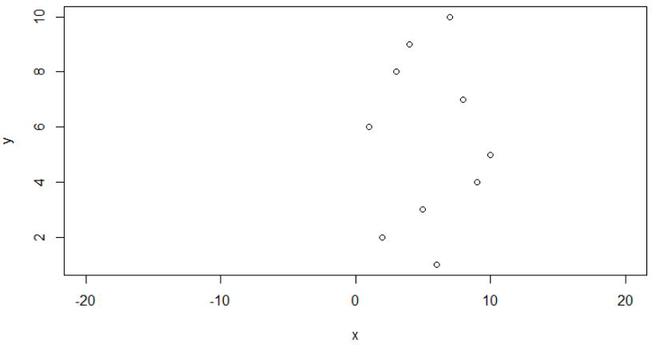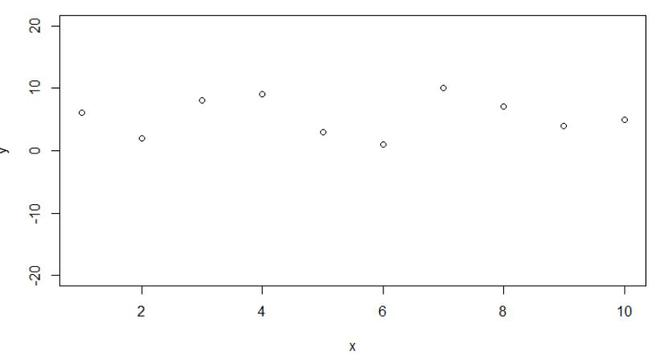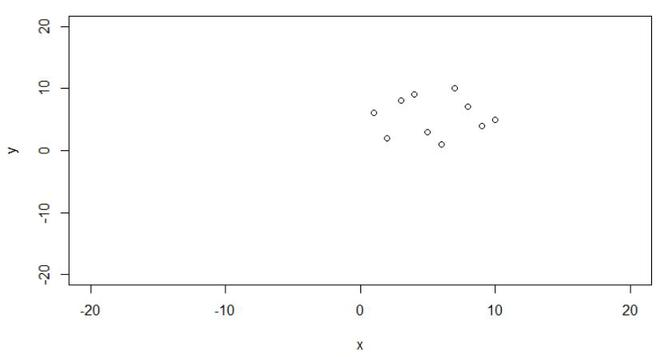# Set Axis Limits of Plot in R

• Last Updated : 23 Aug, 2021

In this article, we will be looking at the approach to set the axis limits of the plot in R programming language.

Axis limit of the plot basically refers to the scaling of the x-axis and the y-axis of the given plot.

## Using xlim and ylim arguments of the plot function

In this approach to set the axis limits of the given plot, the user here just simply use the xlim argument with the required parameters to set the limits of the x-axis limits and use the ylim argument with the required parameters to set the limits of the y-axis limits in the plot function of the R programming language to set the axis limits of the plot given.

Plot function: This is a Generic function for plotting R objects.

Syntax: plot(x, y,xlim,ylim …)

Arguments:

• x: the x coordinates of points in the plot.
• y: the y coordinates of points in the plot.
• xlim: Set or query x-axis limits
• ylim: Set or query y-axis limits

### Example 1: X-Axis Limits Using xlim Argument

In this example, we will be setting up the limits of the x-axis from -20 to 20 of the given plot of 10 data points in it using the xlim argument of the plot function in the R programming language.

## R

 `x = ``c``(4, 9, 5, 6, 10, 2, 3, 7, 8, 1) ` `y = ``c``(9, 4, 3, 1, 5, 2, 8, 10, 7, 6) ` ` `  `plot``(x, y, xlim = ``c``(-20, 20))`

Output:### Example 2: Y-Axis Limits Using ylim Argument

In this example, we will be setting up the limits of the y-axis from -20 to 20 of the given plot of 10 data points in it using the ylim argument of the plot function in the R programming language.

## R

 `x = ``c``(4, 9, 5, 6, 10, 2, 3, 7, 8, 1) ` `y = ``c``(9, 4, 3, 1, 5, 2, 8, 10, 7, 6) ` ` `  `plot``(x, y, ylim = ``c``(-20, 20))`

Output:### Example 3: Set Limits Using xlim and ylim

In this example, we will be setting up the limits of the x-axis and y-axis from -20 to 20 of the given plot of 10 data points in it using the xlim and ylim argument of the plot function in the R programming language.

## R

 `x = ``c``(4, 9, 5, 6, 10, 2, 3, 7, 8, 1) ` `y = ``c``(9, 4, 3, 1, 5, 2, 8, 10, 7, 6) ` ` `  `plot``(x, y, xlim = ``c``(-20, 20), ` `     ``ylim = ``c``(-20, 20)) `

Output:My Personal Notes arrow_drop_up
Recommended Articles
Page :